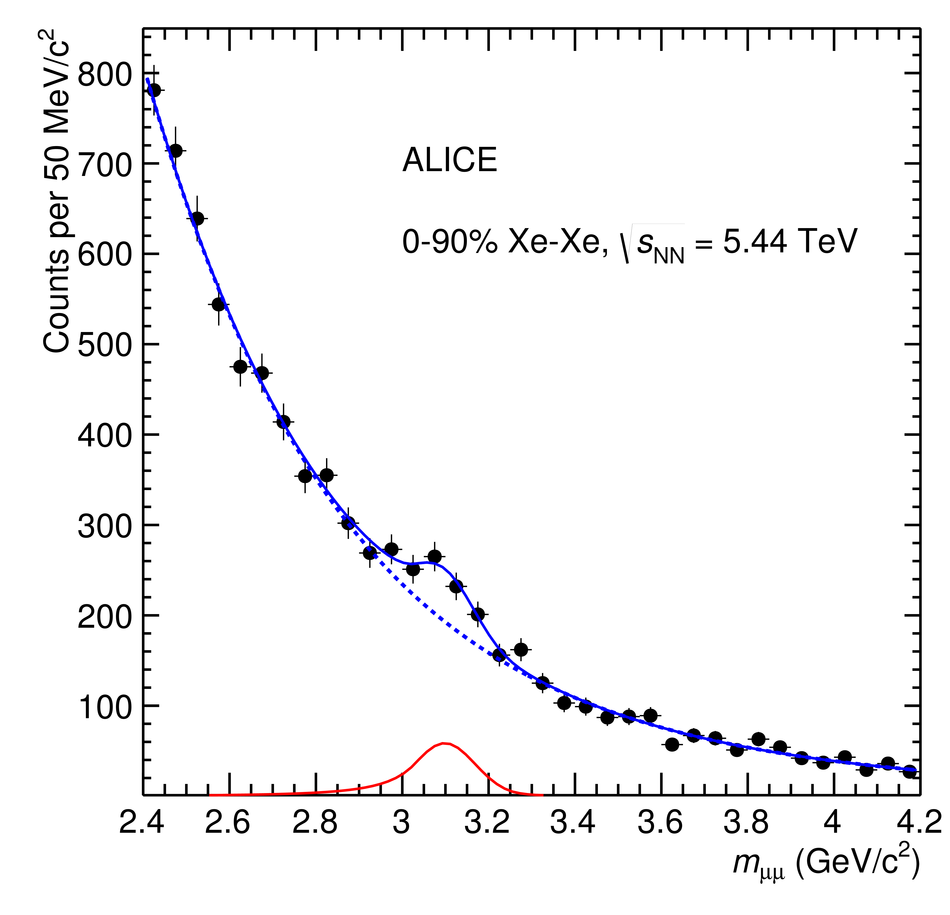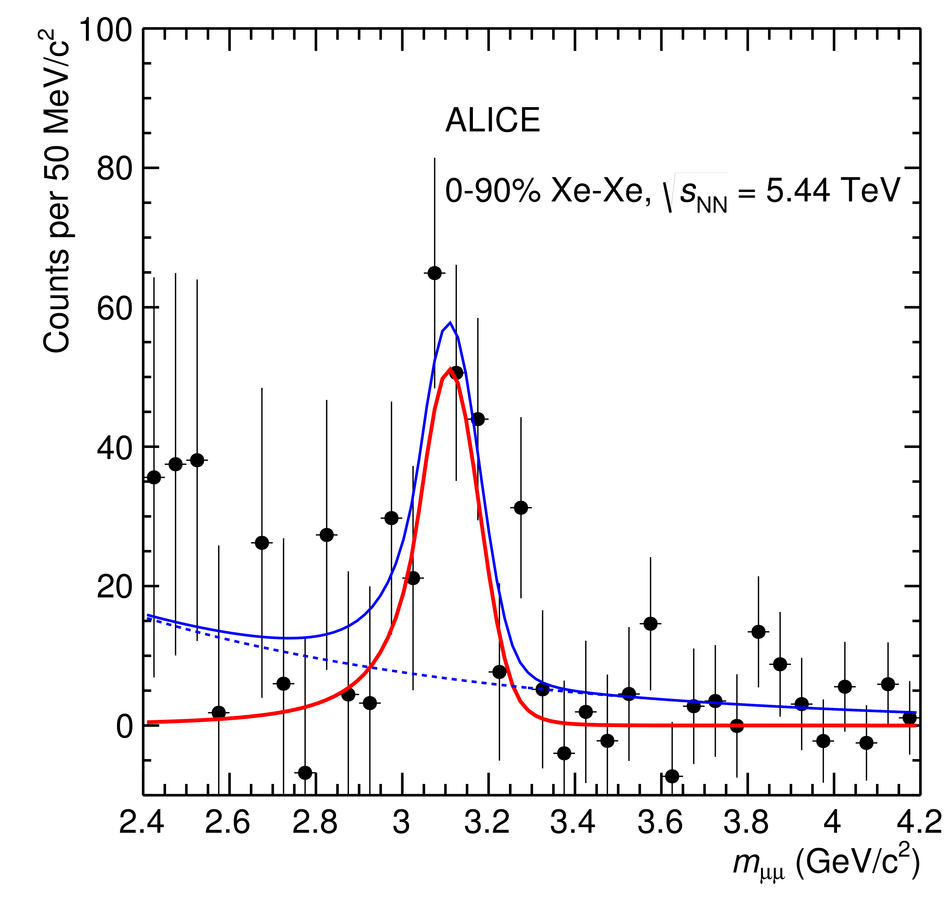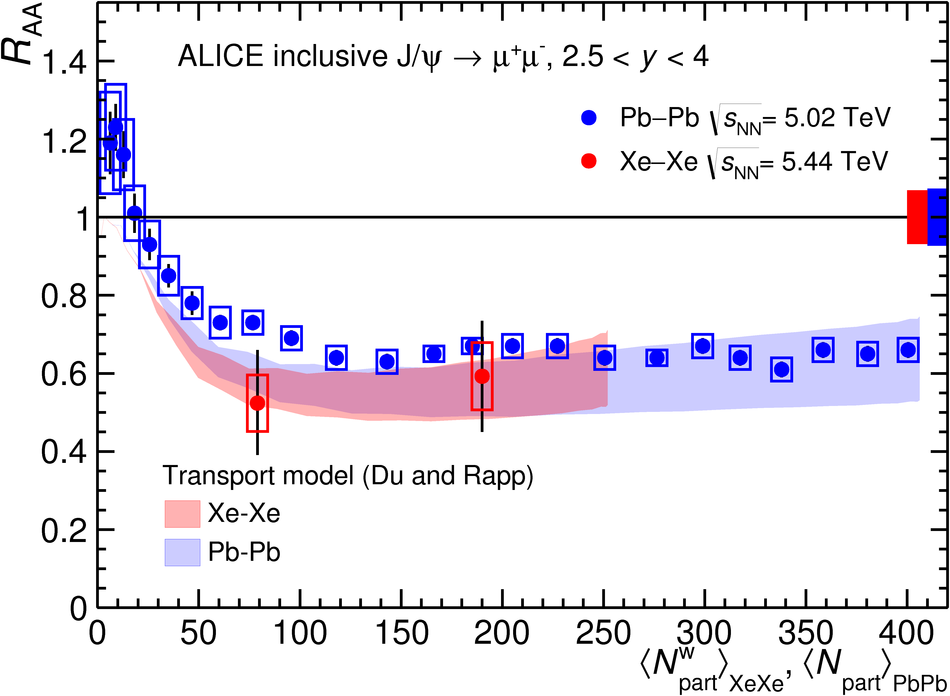Inclusive J/$ψ$ production in Xe-Xe collisions at $\sqrt{s_{\rm NN}}$ = 5.44 TeV

Inclusive J/$\psi$ production is studied in Xe-Xe interactions at a centre-of-mass energy per nucleon pair of $\sqrt{s_{\rm NN}}= 5.44$ TeV, using the ALICE detector at the CERN LHC. The J/$\psi$ meson is reconstructed via its decay into a muon pair, in the centre-of-mass rapidity interval $2.5<~y<~4$ and down to zero transverse momentum. In this Letter, the nuclear modification factors $R_{\rm AA}$ for inclusive J/$\psi$, measured in the centrality range 0-90% as well as in the centrality intervals 0-20% and 20-90% are presented. The $R_{\rm AA}$ values are compared to previously published results for Pb-Pb collisions at $\sqrt{s_{\rm NN}}= 5.02$ TeV and to the calculation of a transport model. A good agreement is found between Xe-Xe and Pb-Pb results as well as between data and the model.

Figures

Figure 1

 Fits to invariant mass distributions of opposite-sign dimuons, for 0--90\% \mbox{Xe--Xe} collisions. In the left panel, the result of a fit to the raw invariant mass spectrum is shown, while in the right panel the fit to the same distribution after subtraction of the mixed-event background is presented. The fit curves shown in blue represent the sum of the signal and background shapes, while the red lines correspond to the J/$\psi$ signal and the blue dashed ones to the background (see text for details).Figure 2

 The inclusive J/$\psi$ nuclear modification factor for \mbox{Xe--Xe} collisions at $\sqrt{s_{\rm NN}}=5.44$ TeV. The results are plotted using as centrality variable $\langle N^{\rm w}_{\rm part}\rangle$, obtained by weighting, in each centrality interval, the $N_{\rm part}$ distribution with the corresponding distribution of the number of nucleon-nucleon collisions. The error bars represent the statistical uncertainties, the boxes around the points the uncorrelated systematic uncertainties. Correlated uncertainties are shown as a filled box around unity The results are compared with the same quantity for \mbox{Pb--Pb} collisions at $\sqrt{s_{\rm NN}}=5.02$ TeV~ and to the results of the calculation of a transport model~. For \mbox{Pb--Pb}, the weighting of $N_{\rm part}$ with the number of nucleon-nucleon collisions was not performed, since it leads to a negligible effect when the centrality intervals are narrow.